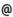## Babes-Bolyai University of Cluj-Napoca Faculty of Mathematics and Computer Science Study Cycle: Graduate SUBJECT

 Code Subject MML0009 Supplement of Algebra
 Section Semester Hours: C+S+L Category Type Mathematics - in Romanian 6 2+1+0 speciality optional Mathematics-Computer Science - in Romanian 6 2+1+0 speciality optional Applied Mathematics 6 2+1+0 speciality optional
 Teaching Staff in Charge
 Assoc.Prof. COVACI Rodica, Ph.D.,  rcovacimath.ubbcluj.ro
 Aims Completion of the knowledge in number theory, group theory and combinatorics studied in the courses of algebra of previous semesters.
 Content 1. Induction. Combinatorics: deduction and induction, mathematical induction (different forms) and Peano's axiomatic study of the set of natural numbers, some applications of the induction; arrangements, permutations and combinations - their general form and applications (number of terms in the canonical form of a polynomial, product of binomials, power of a sum). 2. Arithmetic of numbers and polynomials (comparative study): the division algorithm with remainder - proof for integers and for polynomials. 3. Algebraic structures: an elementary construction of rings and fields, the structure of some finite groups, some applications of finite groups (the symmetry group of a figure, the action of a group on a set, the Polya-Burnside counting method). 4. Algebraic equations: the fundamental theorem of Algebra, complex numbers expressible by radicals, formulae for solving the algebraic equations of second, third and fourth degree.
 References 1. Becheanu, M. si colectiv, Algebra pentru perfectionarea profesorilor, Ed. Didactica si Pedagogica, Bucuresti, 1983. 2. Ion, I.D.; Nastasescu, C.; Nita, C., Complemente de algebra, Ed. Stiintifica si Enciclopedica, Bucuresti, 1984. 3. Kurosh A., Higher Algebra, Mir Publishers, Moscow, 1975. 4. Nastasescu, C.; Nita, C., Teoria calitativa a ecuatiilor algebrice, Editura Tehnica, Bucuresti, 1979. 5. Vraciu C., Vraciu M., Elemente de aritmetica, Editura ALL, Bucuresti, 1998.
 Assessment Exam.
 Links: Syllabus for all subjects Romanian version for this subject Rtf format for this subject